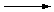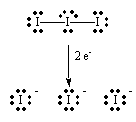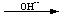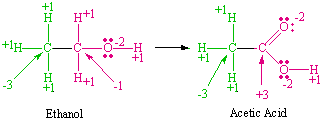Oxidation-Reduction Equations

Oxidation-Reduction Equations

Balancing Oxidation-Reduction Equations

A trial-and-error approach to balancing chemical equations involves playing with the equationadjusting the ratio of the reactants and productsuntil the following goals have been achieved.

 Goals for Balancing Chemical Equations 1. The number of atoms of each element on both sides of the equation is the same and therefore mass is conserved. 2. The sum of the positive and negative charges is the same on both sides of the equation and therefore charge is conserved. (Charge is conserved because electrons are neither created nor destroyed in a chemical reaction.)

There are two situations in which relying on trial and error can get you into trouble. Sometimes the equation is too complex to be solved by trial and error within a reasonable amount of time. Consider the following reaction, for example.

3 Cu(s) + 8 HNO3(aq)3 Cu2+(aq) + 2 NO(g) + 6 NO3-(aq) + 4 H2O(l)

Other times, more than one equation can be written that seems to be balanced. The following are just a few of the balanced equations that can be written for the reaction between the permanganate ion and hydrogen peroxide, for example.

 2 MnO4-(aq) + H2O2(aq) + 6 H+(aq)2 Mn2+(aq) + 3 O2(g) + 4 H2O(l) 2 MnO4-(aq) + 3 H2O2(aq) + 6 H+(aq)2 Mn2+(aq) + 4 O2(g) + 6 H2O(l) 2 MnO4-(aq) + 5 H2O2(aq) + 6 H+(aq)2 Mn2+(aq) + 5 O2(g) + 8 H2O(l) 2 MnO4-(aq) + 7 H2O2(aq) + 6 H+(aq)2 Mn2+(aq) + 6 O2(g) + 10 H2O(l)

Equations such as these have to be balanced by a more systematic approach than trial and error.The Half-Reaction Method of Balancing Redox Equations

A powerful technique for balancing oxidation-reduction equations involves dividing these reactions into separate oxidation and reduction half-reactions. We then balance the half-reactions, one at a time, and combine them so that electrons are neither created nor destroyed in the reaction.

The steps involved in the half-reaction method for balancing equations can be illustrated by considering the reaction used to determine the amount of the triiodide ion (I3-) in a solution by titration with the thiosulfate (S2O32-) ion.

STEP 1: Write a skeleton equation for the reaction. The skeleton equation for the reaction on which this titration is based can be written as follows.

I3- + S2O32-I- + S4O62-

STEP 2: Assign oxidation numbers to atoms on both sides of the equation. The negative charge in the I3- ion is formally distributed over the three iodine atoms, which means that the average oxidation state of the iodine atoms in this ion is -1/3. In the S4O62- ion, the total oxidation state of the sulfur atoms is +10. The average oxidation state of the sulfur atoms is therefore +21/2.

 I3- + S2O32-I- + S4O62- -1/3 +2 -2 -1 +21/2 -2

STEP 3: Determine which atoms are oxidized and which are reduced.STEP 4: Divide the reaction into oxidation and reduction half-reactions and balance these half-reactions one at a time. This reaction can be arbitrarily divided into two half-reactions. One half-reaction describes what happens during oxidation.

 Oxidation: S2O32-S4O62- +2 +21/2

The other describes the reduction half of the reaction.

 Reduction: I3-I- -1/3 -1

It doesn't matter which half-reaction we balance first, so let's start with the reduction half-reaction. Our goal is to balance this half-reaction in terms of both charge and mass. It seems reasonable to start by balancing the number of iodine atoms on both sides of the equation.

 Reduction: I3-3 I-

We then balance the charge by noting that two electrons must be added to an I3- ion to produce 3 I- ions,

 Reduction: I3- + 2 e-3 I-

as can be seen from the Lewis structures of these ions shown in the figure below.We now turn to the oxidation half-reaction. The Lewis structures of the starting material and the product of this half-reaction suggest that we can get an S4O62- ion by removing two electrons from a pair of S2O32- ions, as shown in the figure below.

 Oxidation: 2 S2O32-S4O62- + 2 e-

STEP 5: Combine these half-reactions so that electrons are neither created nor destroyed. Two electrons are given off in the oxidation half-reaction and two electrons are picked up in the reduction half-reaction. We can therefore obtain a balanced chemical equation by simply combining these half-reactions.

 (2 S2O32-S4O62- + 2 e-) + (I3- + 2 e-3 I-) I3- + 2 S2O32-3 I- + S4O62-

STEP 6: Balance the remainder of the equation by inspection, if necessary. Since the overall equation is already balanced in terms of both charge and mass, we simply introduce the symbols describing the states of the reactants and products.

I3-(aq) + 2 S2O32-(aq)3 I-(aq) + S4O62-(aq)Redox Reactions In Acidic Solutions

Some might argue that we don't need to use half-reactions to balance equations because they can be balanced by trial and error. The half-reaction technique becomes indispensable, however, in balancing reactions such as the oxidation of sulfur dioxide by the dichromate ion in acidic solution.

 H+ SO2(aq) + Cr2O72-(aq)SO42-(aq) + Cr3+(aq)

The reason why this equation is inherently more difficult to balance has nothing to do with the ratio of moles of SO2 to moles of Cr2O72-; it results from the fact that the solvent takes an active role in both half-reactions.

 Practice Problem 3:Use half-reactions to balance the equation for the reaction between sulfur dioxide and the dichromate ion in acidic solution. Click here to check your answer to Practice Problem 3. Click here to see a solution to Practice Problem 3.

The reaction between oxalic acid and potassium permanganate in acidic solution is a classical technique for standardizing solutions of the MnO4- ion. These solutions need to be standardized before they can be used because it is difficult to obtain pure potassium permanganate. There are three sources of error.

• Samples of KMnO4 are usually contaminated by MnO2.
• Some of the KMnO4 reacts with trace contaminants when it dissolves in water, even when distilled water is used as the solvent.
• The presence of traces of MnO2 in this system catalyzes the decomposition of MnO4- ion on standing.

Solutions of this ion therefore have to be standardized by titration just before they are used. A sample of reagent grade sodium oxalate (Na2C2O4) is weighed out, dissolved in distilled water, acidified with sulfuric acid, and then stirred until the oxalate dissolves. The resulting oxalic acid solution is then used to titrate MnO4- to the endpoint of the titration, which is the point at which the last drop of MnO4- ion is decolorized and a faint pink color persists for 30 seconds.

 Practice Problem 4:We can determine the concentration of an acidic permanganate ion solution by titrating this solution with a known amount of oxalic acid until the charactistic purple color of the MnO4- ion disappears. H2C2O4(aq) + MnO4-(aq)CO2(g) + Mn2+(aq) Use the half-reaction method to write a balanced equation for this reaction. Click here to check your answer to Practice Problem 4. Click here to see a solution to Practice Problem 4.

Solutions of the MnO4- ion that have been standardized against oxalic acid, using the equation balanced in the previous practice problem, can be used to determine the concentration of aqueous solutions of hydrogen peroxide, using the equation balanced in the following practice problem.

Practice Problem 5:

An endless number of balanced equations can be written for the reaction between the permanganate ion and hydrogen peroxide in acidic solution to form the manganese (II) ion and oxygen:

 MnO4-(aq) + H2O2(aq)Mn2+(aq) + O2(g)

Use the half-reaction method to determine the correct stoichiometry for this reaction.Redox Reactions in Basic Solutions

Half-reactions are also valuable for balancing equations in basic solutions. The key to success with these reactions is recognizing that basic solutions contain H2O molecules and OH- ions. We can therefore add water molecules or hydroxide ions to either side of the equation, as needed.

The following equation describes the reaction between the permanganate ion and hydrogen peroxide in an acidic solution.

2 MnO4-(aq) + 5 H2O2(aq) + 6 H+(aq)2 Mn2+(aq) + 5 O2(g) + 8 H2O(l)

It might be interesting to see what happens when this reaction occurs in a basic solution.

Practice Problem 6:

Write a balanced equation for the reaction between the permanganate ion and hydrogen peroxide in a basic solution to form manganese dioxide and oxygen.

 MnO4-(aq) + H2O2(aq)MnO2(s) + O2(g)

Reactions in which a single reagent undergoes both oxidation and reduction are called disproportionation reactions. Bromine, for example, disproportionates to form bromide and bromate ions when a strong base is added to an aqueous bromine solution.

 OH- Br2Br - + BrO3-

 Practice Problem 7:Write a balanced equation for the disproportionation of bromine in the presence of a strong base. Click here to check your answer to Practice Problem 7. Click here to see a solution to Practice Problem 7.Molecular Redox Reactions

Lewis structures can play a vital role in understanding oxidation-reduction reactions with complex molecules. Consider the following reaction, for example, which is used in the Breathalyzer to determine the amount of ethyl alcohol or ethanol on the breath of individuals who are suspected of driving while under the influence.

3 CH3CH2OH(g) + 2 Cr2O72-(aq) + 16 H+(aq)3 CH3CO2H(aq) + 4 Cr3+(aq) + 11 H2O(l)

We could balance the oxidation half-reaction in terms of the molecular formulas of the starting material and the product of this half-reaction.

 Oxidation: C2H6OC2H4O2

It is easier to understand what happens in this reaction, however, if we assign oxidation numbers to each of the carbon atoms in the Lewis structures of the components of this reaction, as shown in the figure below.The carbon atom in the CH3 group in ethanol is assigned an oxidation state of -3 so that it can balance the oxidation states of the three H atoms it carries. Applying the same technique to the CH2OH group in the starting material gives an oxidation state of -1.

The carbon in the CH3 group in the acetic acid formed in this reaction has the same oxidation state as it did in the starting material: -3. There is a change in the oxidation number of the other carbon atom, however, from -1 to +3. The oxidation half-reaction therefore formally corresponds to the loss of four electrons by one of the carbon atoms.

 Oxidation: CH3CH2OHCH3CO2H + 4 e-

Because this reaction is run in acidic solution, we can add H+ and H2O molecules as needed to balance the equation.

 Oxidation: CH3CH2OH + H2OCH3CO2H + 4 e- + 4 H+

The other half of this reaction involves a six-electron reduction of the Cr2O72- ion in acidic solution to form a pair of Cr3+ ions.

 Reduction: Cr2O72- + 6 e-2 Cr3+

Adding H+ ions and H2O molecules as needed gives the following balanced equation for this half-reaction.

 Reduction: Cr2O72- + 14 H+ + 6 e-2 Cr3+ + 7 H2O

We are now ready to combine the two half-reactions by assuming that electrons are neither created nor destroyed in this reaction.

 3(CH3CH2OH + H2OCH3CO2H + 4 e- + 4 H+) 2(Cr2O72- + 14 H+ + 6 e-2 Cr3+ + 7 H2O) 3 CH3CH2OH + 2 Cr2O72- + 28 H+ + 3 H2O3 CH3CO2H + 4 Cr3+ + 12 H+ + 14 H2O

Simplifying this equation by removing 3 H2O molecules and 12 H+ ions from both sides of the equation gives the balanced equation for this reaction.

3 CH3CH2OH(g) + 2 Cr2O72-(aq) + 16 H+(aq)3 CH3CO2H(aq) + 4 Cr3+(aq) + 11 H2O(l)

 Practice Problem 8:Methyllithium (CH3Li) can be used to form bonds between carbon and either main-group metals or transition metals: HgCl2(s) + 2 CH3Li(l)Hg(CH3)2(l) + 2 LiCl(s) WCl6(s) +  6 CH3Li(l)W(CH3)6(l) + 6 LiCl(s) It can be used also to form bonds between carbon and other nonmetals: PCl3(s) +  3 CH3Li(l)P(CH3)3(l) + 3 LiCl(s) or between carbon atoms: CH3Li(l) + H2CO(g)[CH3CH2OLi]CH3CH2OH(l) Use Lewis structures to explain the stoichiometry of the following oxidation-reaction, which is used to synthesize methyllithium: CH3Br(l) + 2 Li(s)CH3Li(l) + LiBr(s) Click here to check your answer to Practice Problem 8.